# Install necessary packages
list_packages <- c("AER", "dynlm", "fpp", "fpp2",
"quantmod", "urca", "vars", "tseries", "sarima")
new_packages <- list_packages[!(list_packages %in% installed.packages()[,"Package"])]
if(length(new_packages)) install.packages(new_packages)

lapply(list_packages, require, character.only = TRUE)

# 1 Seasonal Data

A time series model exhibits periodic behavior with period $$s$$, if similarities in the time series data occurs after a $$s$$ time interval.

A particular kind of periodic behavior is seasonal behavior in which there are similar “with-in-year” patterns from year to year, e.g., toy sales, airlines usage.

If the basic period is a month, then the period is $$s=12$$. If the data is collected by quarters, then the period is s=4, and there are other possibilities where there can be more than one periodicity, e.g., daily observed data may weakly, monthly, quarterly, yearly patterns.

ARIMA models can be employed successfully to fit and forecast seasonal time series data. The techniques require no new development.

One useful representation of seasonal data is as data from a two way table. Here is an example

Year $$\downarrow$$ + month $$\to$$ Jan Feb Mar … … … … Dec
$$1$$ $$Z_1$$ $$Z_2$$ $$Z_3$$ … … … … $$Z_{12}$$
$$2$$ $$Z_{13}$$ $$Z_{14}$$ $$Z_{15}$$ … … … … $$Z_{24}$$
$$3$$ $$Z_{25}$$ $$Z_{26}$$ $$Z_{27}$$ … … … … $$Z_{36}$$
… … … …
$$r$$ $$Z_{12(r-1)+1}$$ $$Z_{12(r-1)+2}$$ $$Z_{12(r-1)+3}$$ … … … … $$Z_{12r}$$

Within each year (row), the data represents a type of month to month pattern.

Within each month (column), the data represents a type of year to year time pattern.

This is the basic for one useful method for modeling seasonal time series data by multiplicative models. This model attempts to model these two types of patterns separately and then combine both features afterwards.

More specifically, we will build two models: one ARIMA for the column and one ARIMA for the row. We will combine these two models to have seasonal ARIMA.

The assumptions and notations

$$s$$-backward operator: $$B^s$$

$$B^s Z_t = Z_{t-s}$$

$$s$$-difference operator: $$\nabla_s = 1-B^s$$

$$\nabla_s Z_t = Z_t - Z_{t-s}$$

$$\nabla_s^D Z_t = (1-B^s)^D Z_t$$ is the $$D$$-th difference operator on the between period time series data.

# 2 Pure Seasonal ARIMA Models

Denote the $$P$$ AR parameters of this model as $$\Phi_s$$, $$\Phi_{2s}$$,…, and $$\Phi_{Ps}$$, and the autoregressive polynomial is defined as $\Phi_P(B^s)=1-\Phi_s B^s - \Phi_{2s} B^{2s} - ... - \Phi_{Ps} B^{Ps}.$

Denote the $$Q$$ MA parameters of this model as $$\Theta_s$$, $$\Theta_{2s}$$,…, and $$\Theta_{Qs}$$, and the moving average polynomial is defined as $\Theta_Q(B^s)=1-\Theta_s B^s - \Theta_{2s} B^{2s} - ... - \Theta_{Qs} B^{Qs}.$

Let $$\nabla_s^D = (1-B^s)^D$$, then the between period model, i.e., the pure seasonal ARIMA model, can be written as

$\Phi_P(B^s) \nabla_s^D \tilde{Z}_t = \Theta_Q(B^s)a_t$

## 2.1 SARMA$$(p,q)_s$$

When $$D=0$$, we have

$\tilde{Z}_t = \Phi_s \tilde{Z}_{t-s} + \Phi_{2s} \tilde{Z}_{t-2s} ... + \Phi_{Ps} \tilde{Z}_{t-Ps} + a_t - \Theta_s a_{t-s} - \Theta_{2s} a_{t-2s} - ... - \Theta_{2Q} a_{t-2Q}$

## 2.2 SAR$$(1)_{12}$$

$\tilde{Z}_t = \Phi_{12} \tilde{Z}_{t-12} + a_t$

n=200000
ts1 <- sim_sarima(n,model=list(sar=0.8, nseasons=12, sigma2 = 1))
par(mfrow=c(1,3))
acf(ts1,ylim=c(-1,1))
pacf(ts1,ylim=c(-1,1))
plot(ts1[1:100],type="l")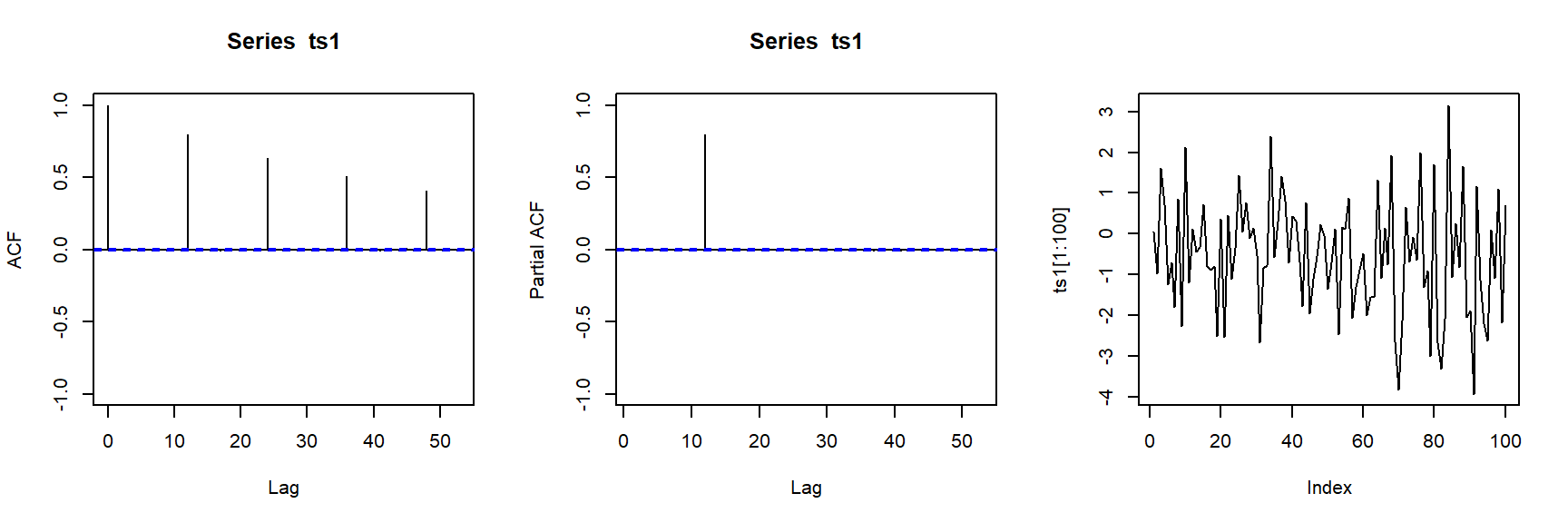## 2.3 SMA$$(1)_{12}$$

$\tilde{Z}_t = a_t - \Theta_{12} a_{t-12}$

n=200000
ts1 <- sim_sarima(n,model=list(sma=0.8, nseasons=12, sigma2 = 1))
par(mfrow=c(1,3))
acf(ts1,ylim=c(-1,1))
pacf(ts1,ylim=c(-1,1))
plot(ts1[1:100],type="l")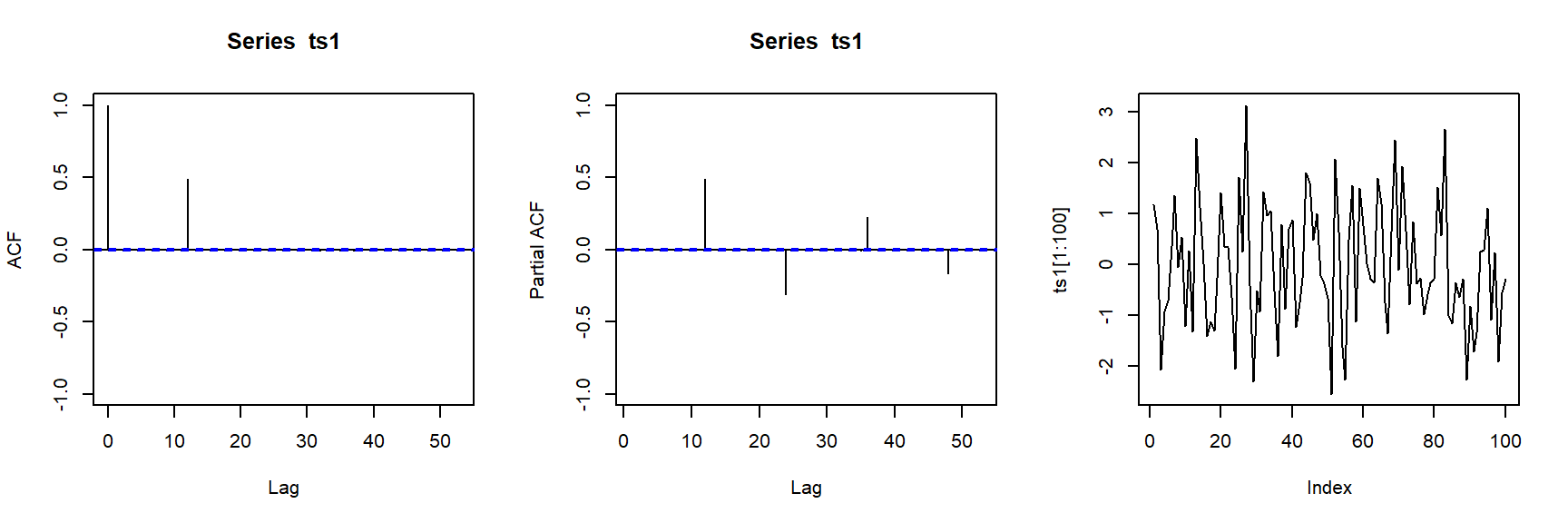# 3 Seasonal ARIMA Models

These pure seasonal models have so far treated the period to period process as an independent process, i.e., no correlation at the lag other than the integer multiple of $$s$$, which is a deficiency.

Therefore, to take consideration of all the dynamics (both between period and within period), we further assume the error component $$a_t$$ are correlated. In fact, we model these $$a_t$$ as another ARIMA(p,d,q), i.e., $$a_t$$ satisfies

$(1-\phi_1B-...-\phi_p B^p)(1-B)^d a_t = (1-\theta_1 B - ... - \theta_q B^q) \epsilon_t$ where $$\epsilon_t$$ is white noise. It can be written as

$\phi(B)(1-B)^d a_t = \theta(B) \epsilon_t$

Or equivalently,

$a_t = \phi^{-1}(B)(1-B)^{-d} \theta(B) \epsilon_t$

Substitute it into the pure seasonal model $$\Phi_P(B^s)\nabla_s^D \tilde{Z}_t = \Theta_Q(B^s)a_t$$, we have the seasonal ARIMA model as

$\Phi_P(B^s)\nabla_s^D \tilde{Z}_t = \Theta_Q(B^s)\phi^{-1}(B)(1-B)^{-d} \theta(B) \epsilon_t$

which is equivalent to

$\Phi_P(B^s) \phi(B) (1-B^s)^D (1-B)^{d} \tilde{Z}_t = \Theta_Q (B^s) \theta(B) \epsilon_t$

This is called the Box-Jenkins Seasonal Multiplicative Model of order $$(p,d,q)\times (P,D,Q)_s$$.

The nonseasonal part (within period) is governed by $$(p,d,q)$$ with AR in $$p$$ and MA in $$q$$ and number of differencing in $$d$$, $$Z_t-Z_{t-1}$$. The seasonal part (between period) is governed by $$(P,D,Q)_s$$ with seasonal AR in $$P$$ and seasonal MA in $$Q$$ and the number of seasonal differencing in $$D$$, $$Z_t-Z_{t-s}$$.

## 3.1 SARIMA$$(0,0,1) \times (1,0,0)_{12}$$

$(1-\Phi_{12} B^{12}) \tilde{Z}_t = a_t - \Theta_{12} a_{t-12}$

n=200000
ts1 <- sim_sarima(n,model=list(ma=0.4, sar=0.8, nseasons=12, sigma2 = 1))
par(mfrow=c(1,3))
acf(ts1,ylim=c(-1,1))
pacf(ts1,ylim=c(-1,1))
plot(ts1[1:100],type="l")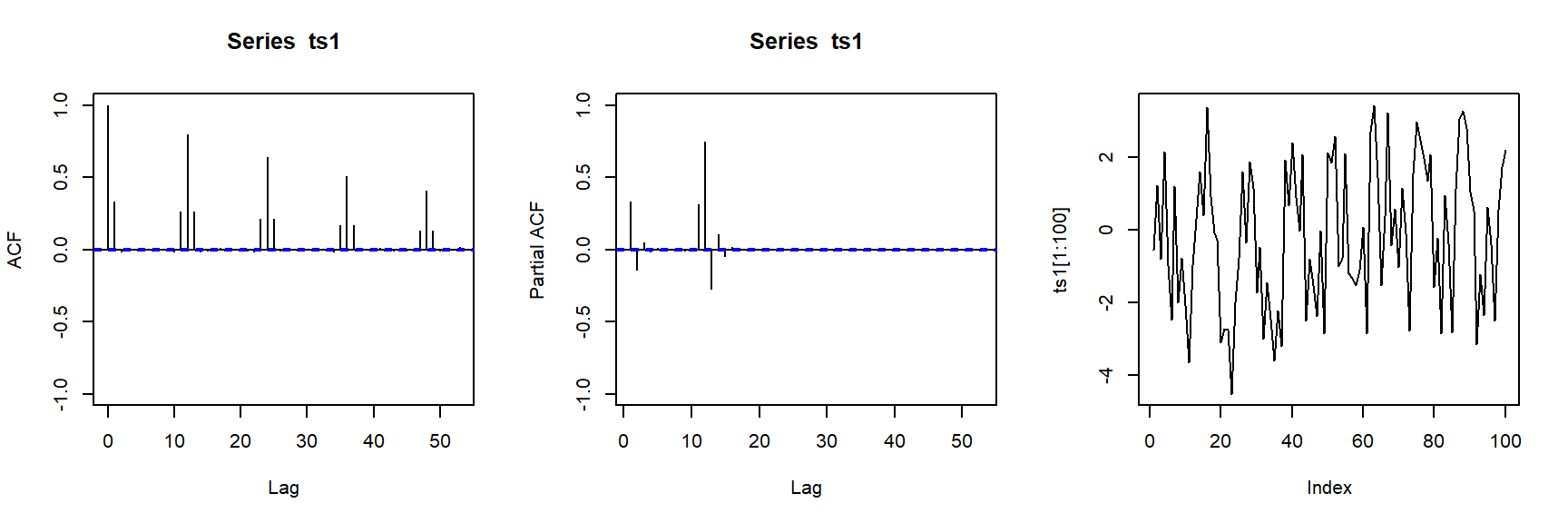## 3.2 SARIMA$$(1,0,0) \times (1,0,0)_{12}$$

$(1-\phi_{1} B) (1-\Phi_{12} B^{12})\tilde{Z}_t = a_t$

n=200000
ts1 <- sim_sarima(n,model=list(ar=0.4, sar=0.8, nseasons=12, sigma2 = 1))
par(mfrow=c(1,3))
acf(ts1,ylim=c(-1,1))
pacf(ts1,ylim=c(-1,1))
plot(ts1[1:100],type="l")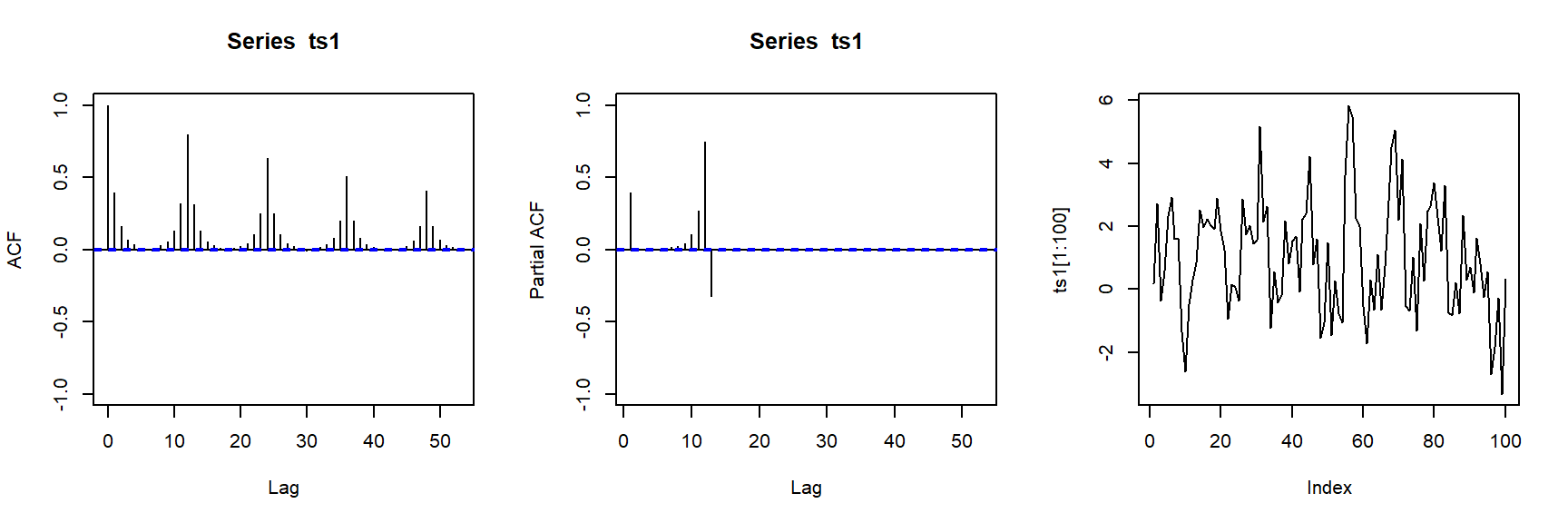It can be proved that the ACF is symmetric around period intervals, i.e., $$\rho_{s+1}=\rho_{s-1}$$.

## 3.3 SARIMA$$(0,0,1) \times (0,0,1)_{12}$$

$\tilde{Z}_t = (1-\Theta_{12} B^{12})(1-\theta_{1}B)a_t$

n=200000
ts1 <- sim_sarima(n,model=list(ma=0.4, sma=0.8, nseasons=12, sigma2 = 1))
par(mfrow=c(1,3))
acf(ts1,ylim=c(-1,1))
pacf(ts1,ylim=c(-1,1))
plot(ts1[1:100],type="l")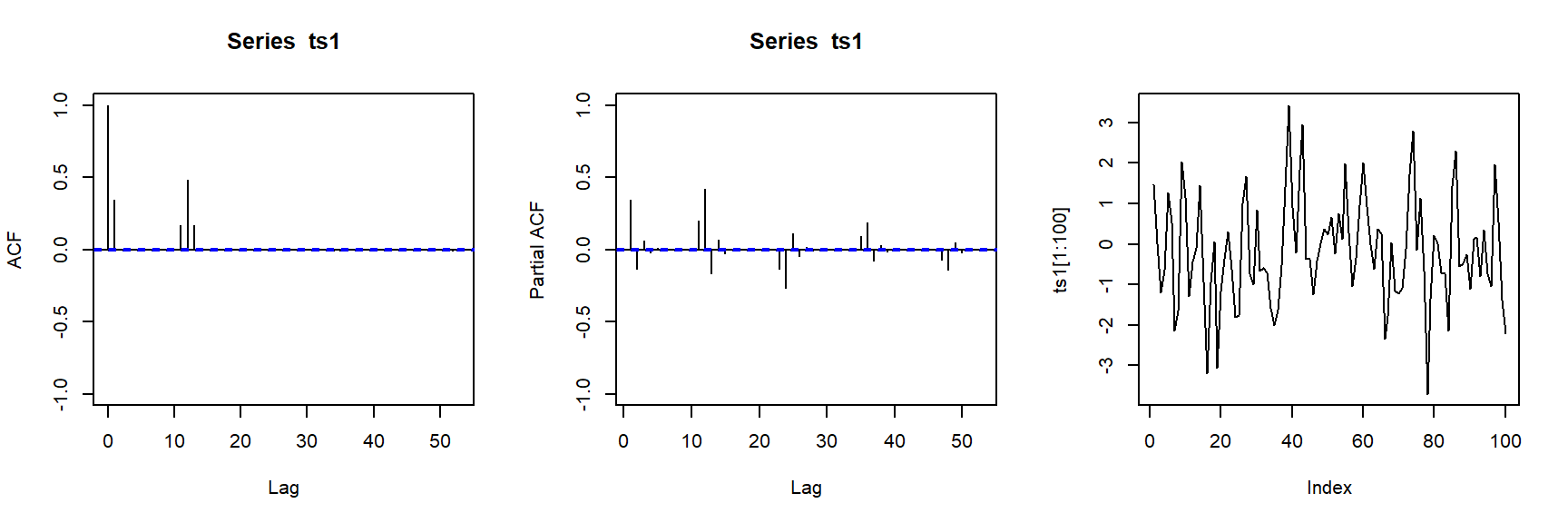## 3.4 SARIMA$$(1,0,0) \times (0,0,1)_{12}$$

$\tilde{Z}_t = a_t - \Theta_s a_{t-s}$

n=200000
ts1 <- sim_sarima(n,model=list(ar=0.4, sma=0.8, nseasons=12, sigma2 = 1))
par(mfrow=c(1,3))
acf(ts1,ylim=c(-1,1))
pacf(ts1,ylim=c(-1,1))
plot(ts1[1:100],type="l")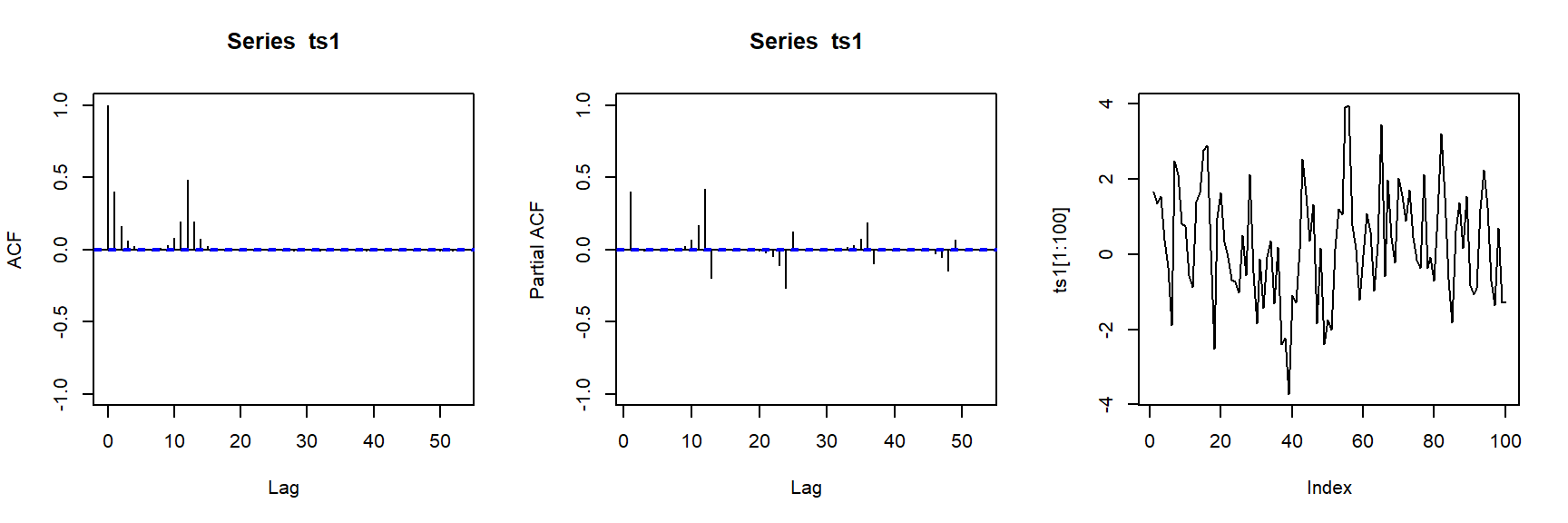## 3.5 SARIMA$$(0,1,0) \times (1,0,0)_{12}$$

$(1-\phi_1 B )(1-B^{12})\tilde{Z}_t = a_t$

n=200000
ts1 <- sim_sarima(n,model=list(sar=0, ar=0.5, siorder=1, nseasons=12, sigma2 = 1))
par(mfrow=c(1,3))
acf(ts1,ylim=c(-1,1))
pacf(ts1,ylim=c(-1,1))
plot(ts1[1:100],type="l")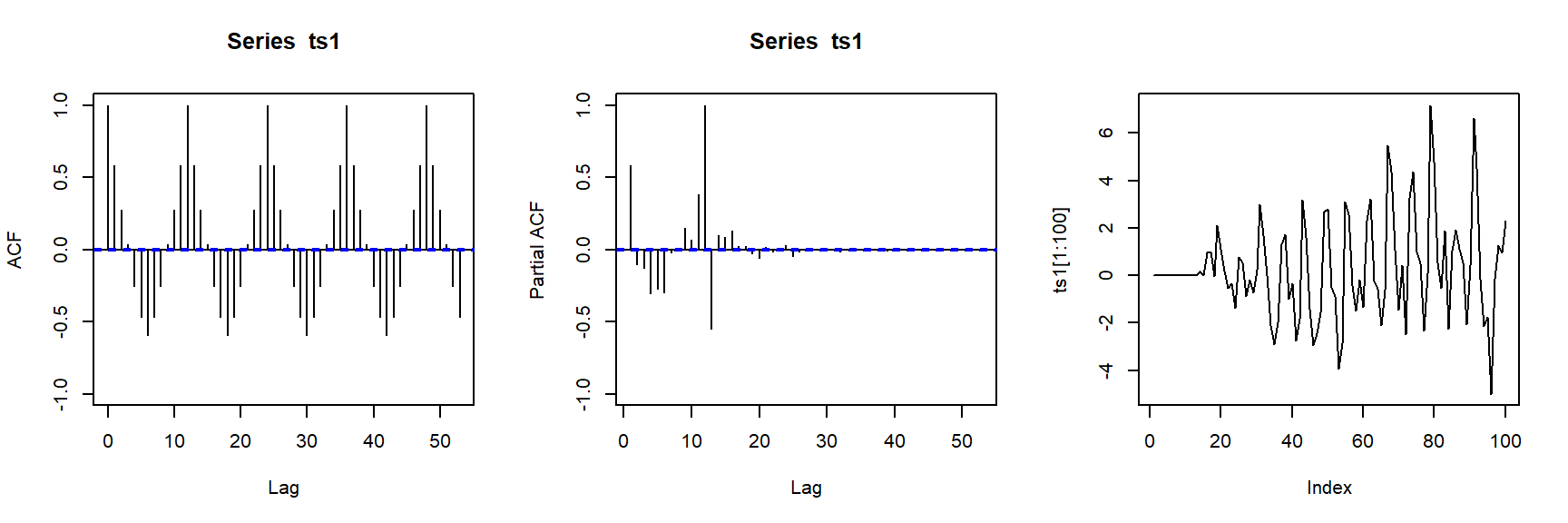## 3.6 SARIMA$$(0,1,0) \times (0,1,0)_{12}$$

$(1- B )(1-B^{12})\tilde{Z}_t = a_t$

n=200000
ts1 <- sim_sarima(n,model=list(iorder=1, siorder=1, nseasons=12, sigma2 = 1))
par(mfrow=c(1,3))
acf(ts1,ylim=c(-1,1))
pacf(ts1,ylim=c(-1,1))
plot(ts1[1:100],type="l")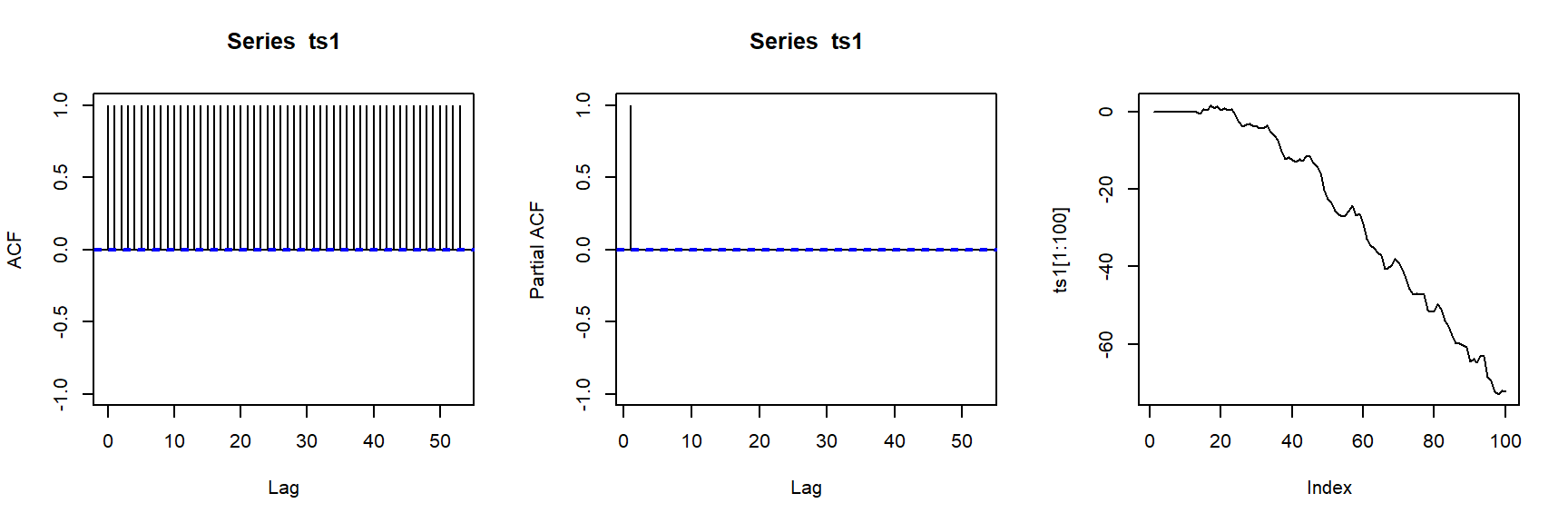## 3.7 SARIMA$$(1,0,0) \times (1,0,0)_{12}$$

$(1- \phi_1 B )(1- \Phi_{12} B^{12})\tilde{Z}_t = a_t$

n=200000
ts1 <- sim_sarima(n,model=list(sar=0.9,ar=0.9, nseasons=12, sigma2 = 1))
par(mfrow=c(1,3))
acf(ts1,ylim=c(-1,1))
pacf(ts1,ylim=c(-1,1))
plot(ts1[1:100],type="l")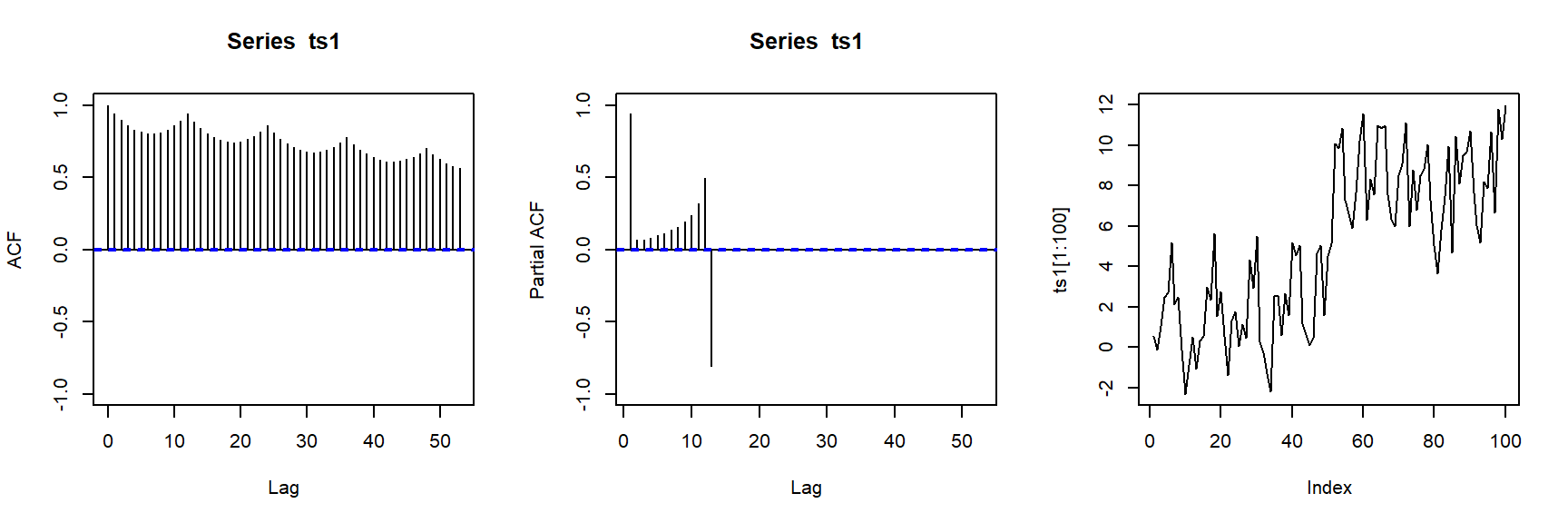These examples give us some intuition of the ACF of seasonal ARIMA.

# 4 Diagnostic Checking, Forecast, and Stationarity

Seasonal ARIMA presents no new problems in terms of diagnostic checking. We simply check adequacy of the a ARIMA model.

Forecast also presents no new challenges.

The condition of stationarity and invertibility for seasonal ARIMA is a direct extension of regular ARIMA. Namely, the seasonal ARIMA is stationary if the roots of both $$\phi_p(B)=0$$ and $$\Phi_P(B^s)=0$$ lie outside of unit circle. The seasonal ARIMA is invertible if the roots of both $$\theta_q(B)=0$$ and $$\Theta_Q(B^s)=0$$ lie outside of unit circle.

# 5 Real Data Examples

Case 9: air-carrier freight

case9=ts(scan("case9.txt"), start=c(1969,1), end=c(1978,12), frequency=12)
case9
##       Jan  Feb  Mar  Apr  May  Jun  Jul  Aug  Sep  Oct  Nov  Dec
## 1969 1299 1148 1345 1363 1374 1533 1592 1687 1384 1388 1295 1489
## 1970 1403 1243 1466 1434 1520 1689 1763 1834 1494 1439 1327 1554
## 1971 1405 1252 1424 1517 1483 1605 1775 1840 1573 1617 1485 1710
## 1972 1563 1439 1669 1651 1654 1847 1931 2034 1705 1725 1687 1842
## 1973 1696 1534 1814 1796 1822 2008 2088 2230 1843 1848 1736 1826
## 1974 1766 1636 1921 1882 1910 2034 2047 2195 1765 1818 1634 1818
## 1975 1698 1520 1820 1689 1775 1968 2110 2241 1803 1899 1762 1901
## 1976 1839 1727 1954 1991 1988 2146 2301 2338 1947 1990 1832 2066
## 1977 1952 1747 2098 2057 2060 2240 2425 2515 2128 2255 2116 2315
## 1978 2143 1948 1460 2344 2363 2630 2811 2972 2515 2536 2414 2545
case9 %>% ggtsdisplay(lag.max=40)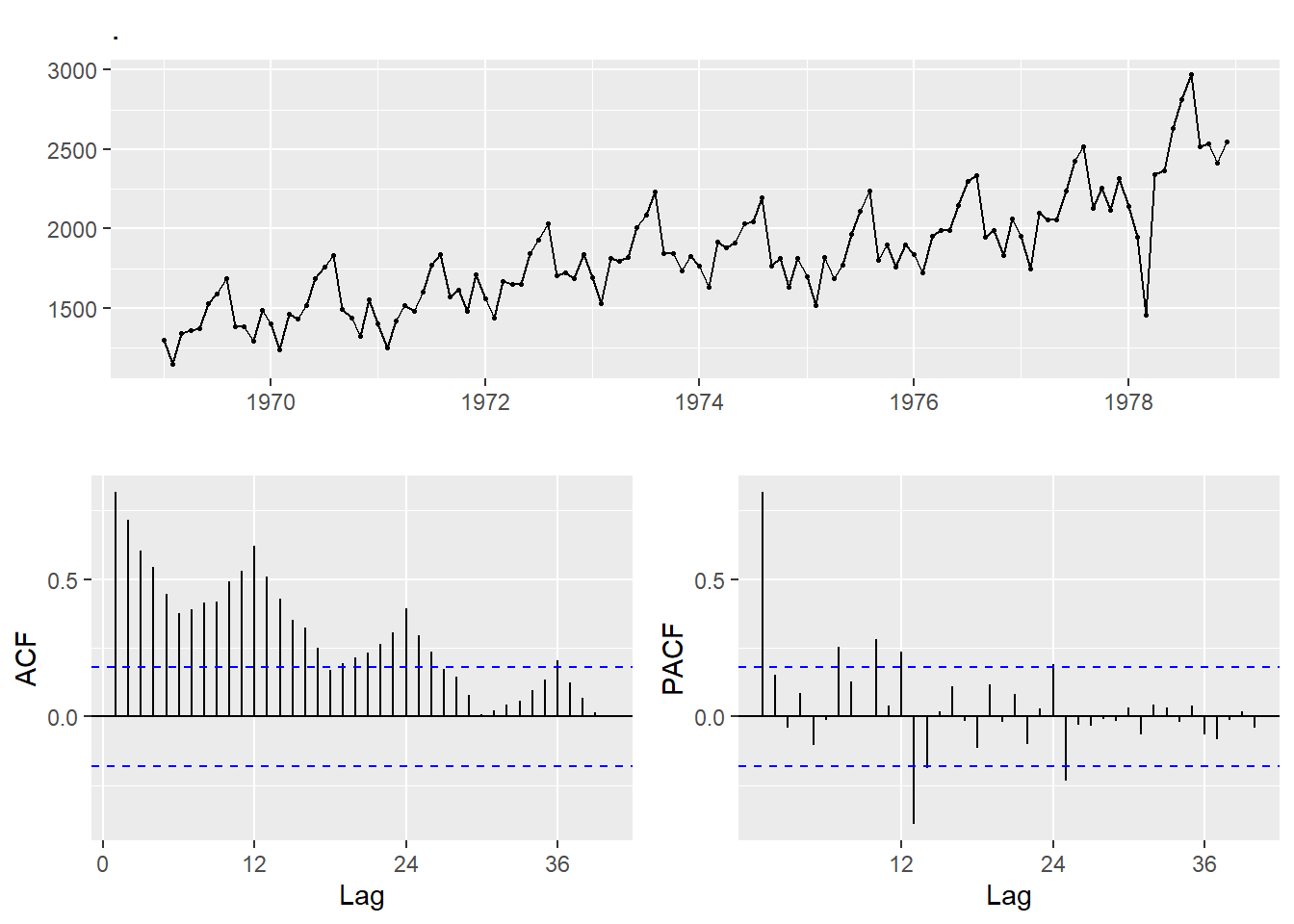Visual check finds the variance increases as time goes by, therefore, we use box-cox transformation with lambda=0, i.e., log transformation.

case9 %>% log() %>% ggtsdisplay(lag.max=40)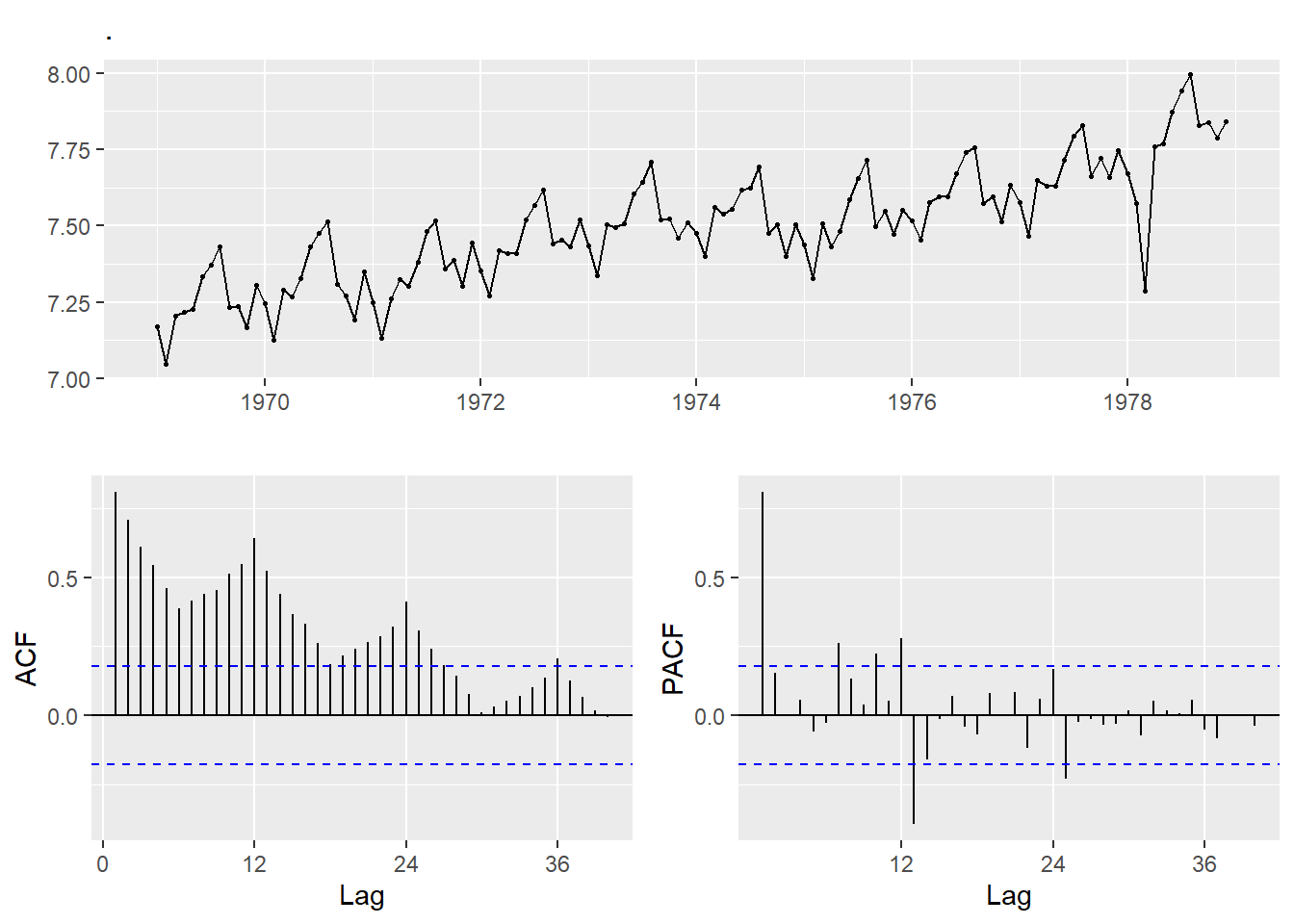The variance is stablized. Because of the ACF, we consider it to be nonstationary and take a 1st order difference.

case9 %>% log() %>% diff() %>% ggtsdisplay(lag.max=40)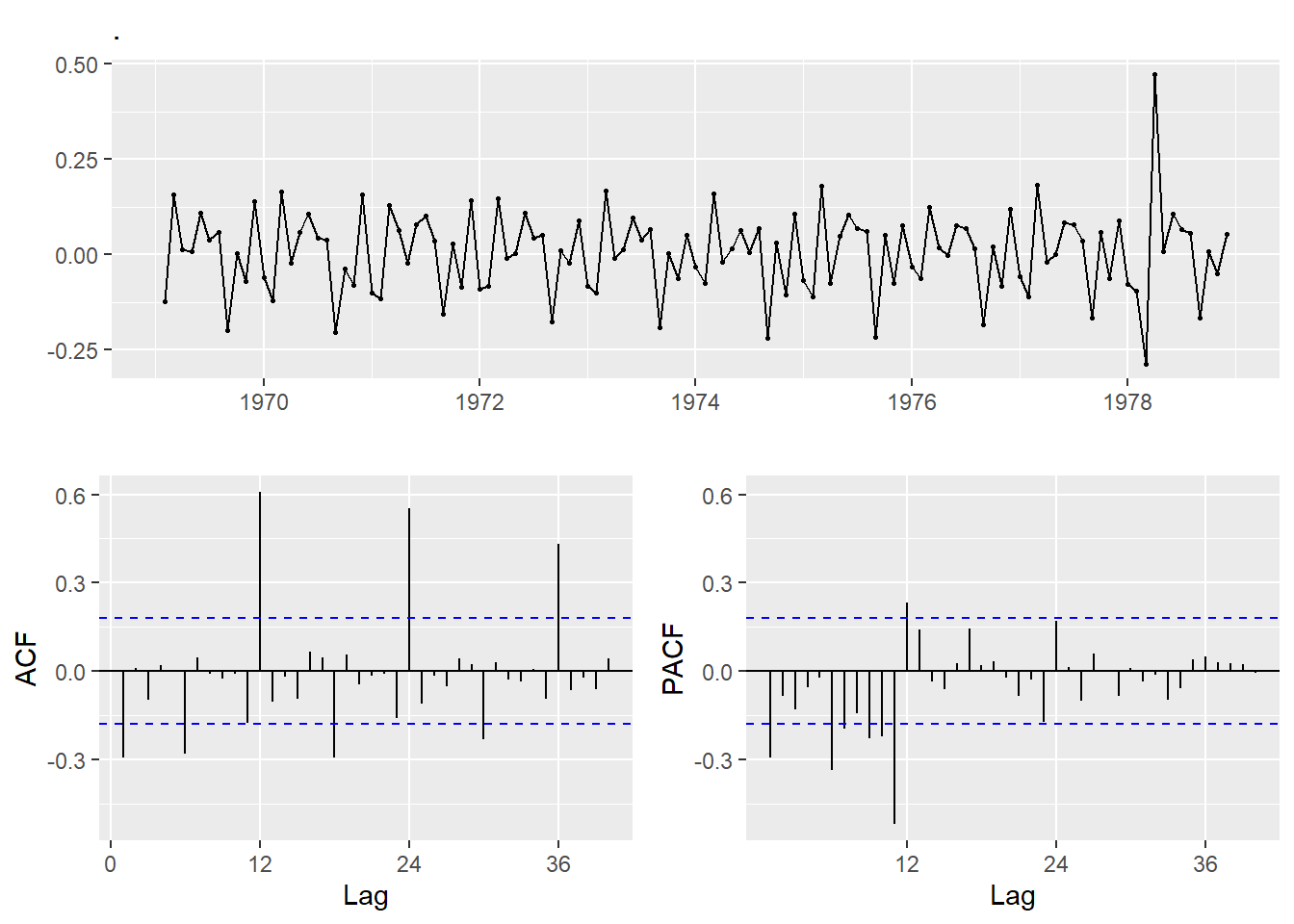The ACF at lags 12, 24, 36 are still decreasing slowly, therefore, we take a seasonal 1st order difference.

case9 %>% log() %>% diff() %>% diff(lag=12) %>% ggtsdisplay(lag.max=40)